Finite element contact modeling method and error characteristics of partitioned parallel computations for bullet penetration simulations
, LIU Sai
Department of Automotive Engineering, Tsinghua University, Beijing 100084, China
Abstract: Improved ballistic simulations were developed for homogeneous steel sheets against small-caliber bullets. The simulations analyzed the influences of the contact-force penalty function parameters and contact friction coefficients on the contact interface state and ballistic limit velocity. The inconsistencies among computed ballistic limit velocities predicted by different partitioned parallel systems were investigated statistically. The interface stiffnesses within the contact-force penalty function should be defined between different contact pairs rather than assigning an equal interface stiffness to all contact regions to improve the accuracy of the ballistic limit velocity. Different friction coefficients between contact pairs can seriously affect the computed ballistic limit velocity for thicker steel targets against armor piercing bullets. Further study is needed to determine the correct friction coefficients for multiple contact pairs. The exchange of regional data and the use of this data in different sequences make the partitioned parallel computations of repeated computation tasks inconsistent, which affects the ballistic limit velocities predicted by the parallel computations. Therefore, repeated calculations using the same model for the same impact velocity are needed to obtain reliable ballistic limit velocity statistics.
Key words: bullet penetration     finite element analysis     contact-force penalty method     contact friction coefficient     ballistic limit velocity     partitioned parallel computation error

1 有限元接触力罚函数算法对弹道极限速度计算值的影响 1.1 基于接触力罚函数算法的穿入修正原理

 ${F_i} =-{F_j} =-k{\cdot}\Delta {x_{ij}}.$ (1)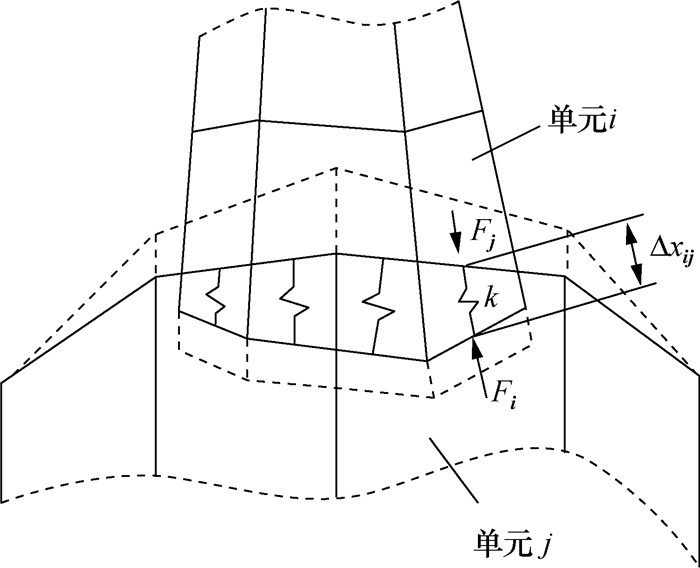图 1 接触力罚函数算法示意图

 $k = s{\cdot}\frac{{K{A^2}}}{V},$ (2)

 $k = 0.5{\cdot}s{\cdot}\left( {\frac{{{m_1}{m_2}}}{{{m_1} + {m_2}}}} \right)\frac{1}{{{{(\Delta t)}^2}}}.$ (3)

 $\Delta x_{ij}^r = \sum\limits_{q = 1}^r {x_{ij}^q},$ (4)
 $\begin{array}{l} W_{_{ij}}^{^r} = W_{_{ij}}^{^{r-1}} + \left( {F_{_i}^{^r}x_{_i}^{^r} + F_{_j}^{^r}x_{_j}^{^r}} \right) = W_{_{ij}}^{^{r-1}} + F_{_i}^{^r}x_{_{ij}}^{^r} = \\ W_{_{ij}}^{^{r-1}} - k\Delta x_{_{ij}}^{^{r - 1}}x_{_{ij}}^{^r} = W_{_{ij}}^{^{r - 1}} - k\left( {\sum\limits_{q = 1}^{r-1} {x_{ij}^q} } \right)x_{_{ij}}^{^r}. \end{array}$ (5)

 $|\Delta x_{_{\mathit{\Phi}} }^{^r}| = \mathop {{\rm{max}}}\limits_{\left( {i, j} \right) \in {\mathit{\Phi}} } |\Delta x_{_{ij}}^{^r}|,$ (6)
 $W_{\mathit{\Phi}} ^r = \sum\limits_{(i, j) \in {\mathit{\Phi}} } {W_{ij}^r.}$ (7)
 $\begin{array}{l} |\Delta {x_{\mathit{\Phi}} }{|_{{\rm{max}}}} = \\ {\rm{max}}\{ |\Delta x_{_{\rm{{\mathit{\Phi}} }}}^{^m}|, |\Delta x_{_{\mathit{\Phi}} }^{^{m + 1}}|, |\Delta x_{_{\mathit{\Phi}} }^{^{m + 2}}|, {\cdots}, |\Delta x_{_{\mathit{\Phi}} }^{^n}|\}, \end{array}$ (8)
 $\begin{array}{l} {\left| {{W_{\mathit{\Phi}} }} \right|_{{\rm{max}}}} = \\ {\rm{max}}\left\{ {\left| {W_{_{\mathit{\Phi}} }^{^m}} \right|, \left| {W_{_{\mathit{\Phi}} }^{^{m + 1}}} \right|, \left| {W_{_{\mathit{\Phi}} }^{^{m + 2}}} \right|, {\cdots}, |W_{_{\mathit{\Phi}} }^{^n}|} \right\}. \end{array}$ (9)

1.2 接触力罚函数算法的接触刚度参数优化设置方法对弹道极限速度计算值的影响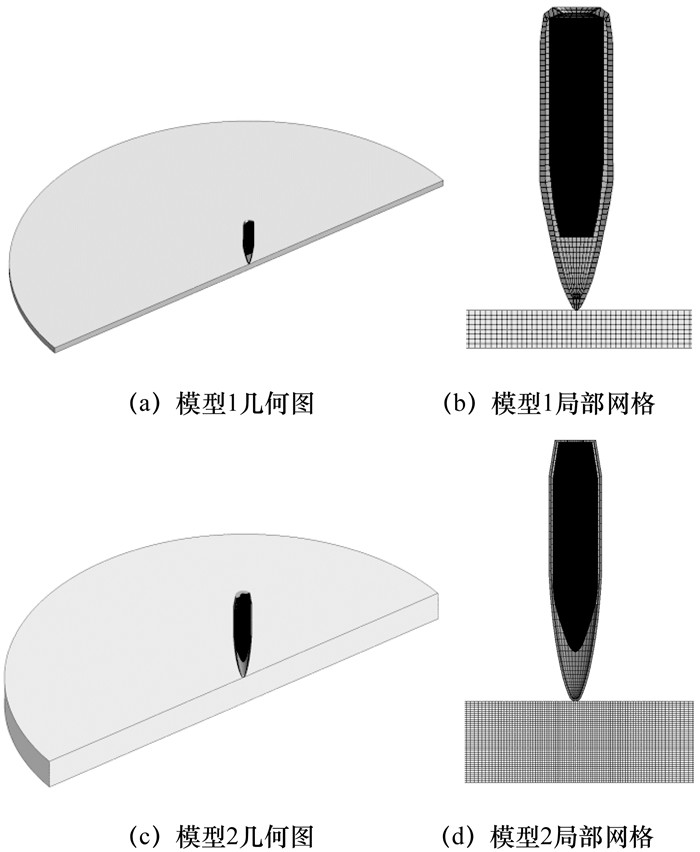图 2 小口径枪弹侵彻均质钢靶板的模型剖视图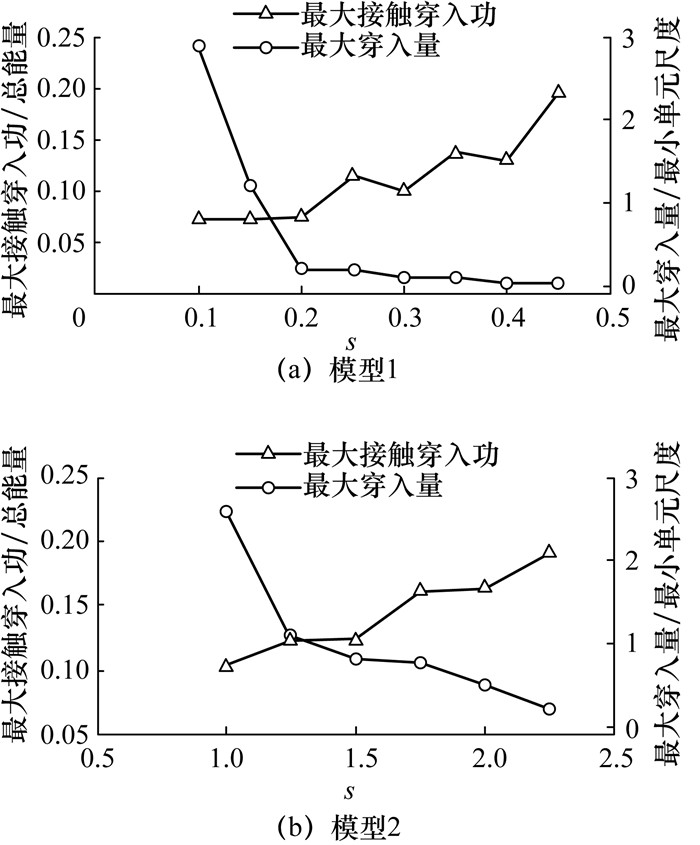图 3 接触刚度系数对最大接触穿入功和最大穿入量的影响

 弹芯与铅套 靶板与铅套 弹芯与靶板 弹芯与被甲 靶板与被甲 被甲与铅套 模型1 0.04 0.4 0.2 0.05 0.2 0.01 模型2 0.05 0.2 2.0 0.3 1.5 0.02

2 有限元模型的接触摩擦系数对弹道极限速度计算值的影响图 4 接触摩擦系数对弹道极限速度计算值的影响

 弹芯与铅套 靶板与铅套 弹芯与靶板 弹芯与被甲 靶板与被甲 被甲与铅套 模型1 0.03 0.4 0.2 0.05 0.2 0.01 模型2 0.08 0.3 3.5 0.3 2.0 0.02

 摩擦系数 靶板破坏形式 磨蚀部分的能量/总能量 摩擦功/总能量 % % 模型1 0.01 花瓣型 25 0.9 0.05 花瓣型 26 3.7 模型2 0.01 扩孔型 54 2.2 0.05 扩孔型 53 8.9

3 弹道冲击过程仿真分析的分区并行计算图 5 模型1的2核并行计算区域分割

 序号 1 2 3 4 5 6 7 8 9 10 11 12 13 14 15 16 17 18 19 20 结果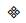⊙ ⊙⊙⊙⊙ ⊙ ⊙⊙ ⊙⊙ 注：⊙表示临界穿透，表示未穿透

 数值实验次数 10 12 14 16 18 20 穿透频率 0.4 0.5 0.5 0.44 0.5 0.5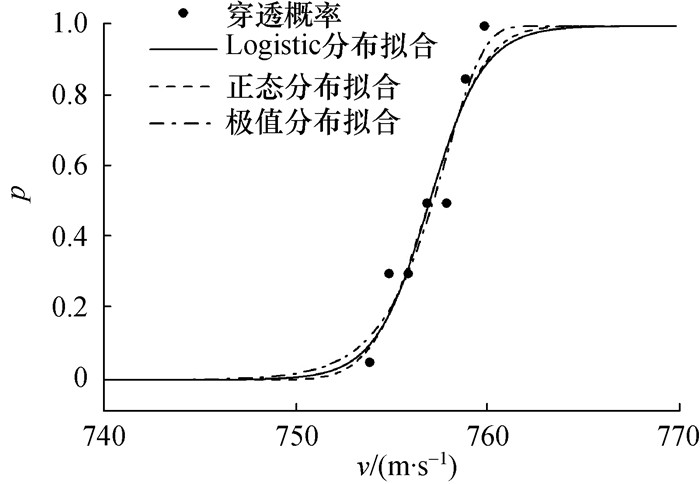图 6 2核并行计算的穿透概率分布(模型1)

 ${p_{\rm{L}}}\left( v \right) = \frac{{{\rm{exp}}\left( {\frac{{v-\mu }}{\sigma }} \right)}}{{\sigma {{\left( {1 + {\rm{exp}}\left( {\frac{{v-\mu }}{\sigma }} \right)} \right)}^2}}},$ (10)
 ${p_{\rm{N}}}\left( v \right) = \frac{1}{{\sigma \sqrt {2\pi } }}{\rm{exp}}\left( {\frac{{-{{\left( {v-\mu } \right)}^2}}}{{2{\sigma ^2}}}} \right),$ (11)
 ${p_{{\rm{EV}}}}\left( v \right) = {\sigma ^{-1}}{\rm{exp}}\left( {\frac{{v-\mu }}{\sigma }} \right){\rm{exp}}\left( {-{\rm{exp}}\left( {\frac{{v - \mu }}{\sigma }} \right)} \right).$ (12)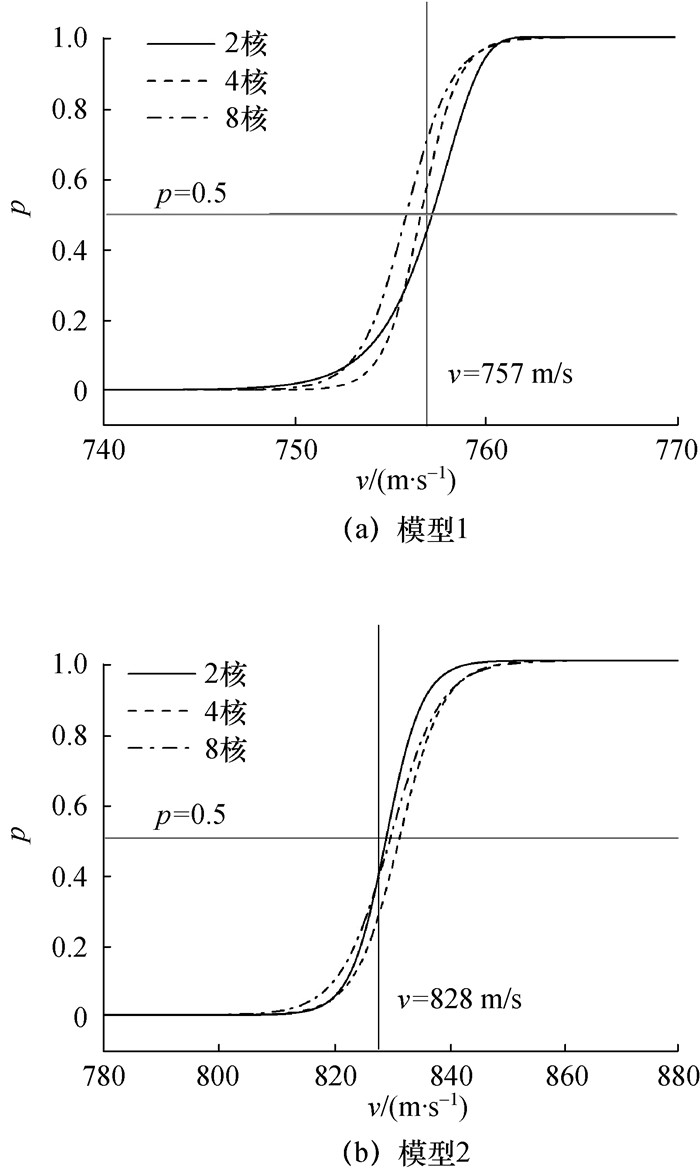图 7 多核并行计算的穿透概率分布

 并行计算系统 速度区间/(m·s-1) (速度差/弹道极限速度值)/% 模型1 2核 752.2~760.2 1.1 4核 753.8~759.6 0.8 8核 752.4~759.5 0.9 模型2 2核 820.1~838.5 2.2 4核 820.3~842.9 2.7 8核 817.1~842.9 3.1

 弹道极限速度统计值/ (m·s-1) 单核 2核 4核 8核 模型1 757 757.3 756.7 755.9 模型2 828 829.3 831.6 830.0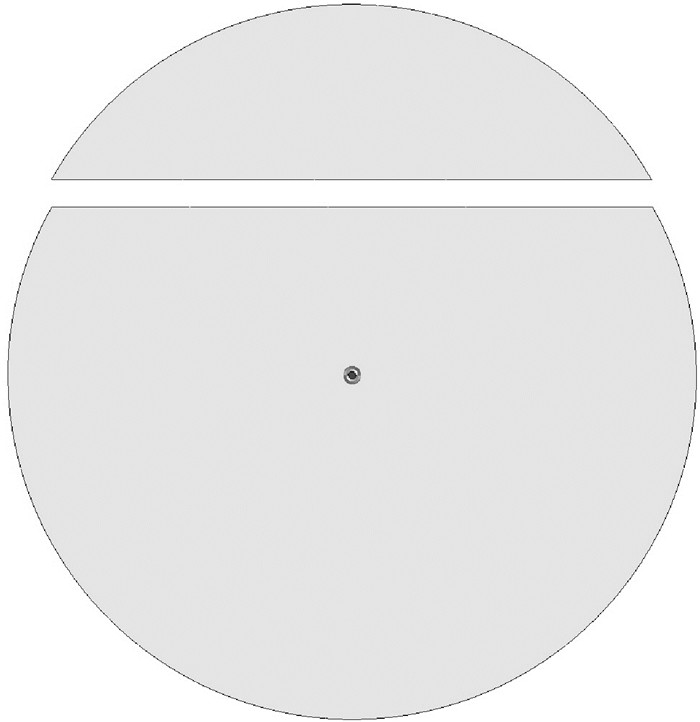图 8 模型1的2核并行计算的非对称区域分割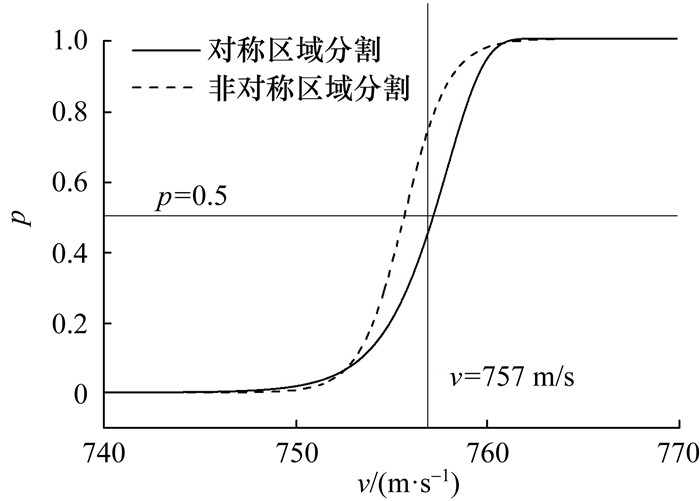图 9 非对称区域分割的2核并行计算的穿透概率分布

4 结束语

  Hallquist J O, Goudreau G L, Benson D J. Sliding interfaces with contact-impact in large-scale Lagrangian computations[J]. Computer Methods in Applied Mechanics and Engineering, 1985, 51(1-3): 107–137. DOI:10.1016/0045-7825(85)90030-1  Peric D, Owen D R J. Computational model for 3-D contact problems with friction based on the penalty method[J]. International Journal for Numerical Methods in Engineering, 1992, 35(6): 1289–1309. DOI:10.1002/(ISSN)1097-0207  BLørvik T, Olovsson L, Dey S, et al. Normal and oblique impact of small arms bullets on AA6082-T4 aluminium protective plates[J]. International Journal of Impact Engineering, 2011, 38(7): 577–589. DOI:10.1016/j.ijimpeng.2011.02.001  Holmen J K, Johnsen J, Jupp S, et al. Effects of heat treatment on the ballistic properties of AA6070 aluminium alloy[J]. International Journal of Impact Engineering, 2013, 57: 119–133. DOI:10.1016/j.ijimpeng.2013.02.002  BLørvik T, Dey S, Olovsson L. Penetration of granular materials by small-arms bullets[J]. International Journal of Impact Engineering, 2015, 75: 123–139. DOI:10.1016/j.ijimpeng.2014.07.016  Jankowiak T, Rusinek A, Wood P. A numerical analysis of the dynamic behaviour of sheet steel perforated by a conical projectile under ballistic conditions[J]. Finite Elements in Analysis and Design, 2013, 65: 39–49. DOI:10.1016/j.finel.2012.10.007  Kpenyigba K M, Jankowiak T, Rusinek A, et al. Influence of projectile shape on dynamic behavior of steel sheet subjected to impact and perforation[J]. Thin-Walled Structures, 2013, 65: 93–104. DOI:10.1016/j.tws.2013.01.003  Arias A, Rodríguez-Martínez J A, Rusinek A. Numerical simulations of impact behaviour of thin steel plates subjected to cylindrical, conical and hemispherical non-deformable projectiles[J]. Engineering Fracture Mechanics, 2008, 75(6): 1635–1656. DOI:10.1016/j.engfracmech.2007.06.005  Rusinek A, Rodríguez-Martínez J A, Arias A, et al. Influence of conical projectile diameter on perpendicular impact of thin steel plate[J]. Engineering Fracture Mechanics, 2008, 75(10): 2946–2967. DOI:10.1016/j.engfracmech.2008.01.011  Farhat C. A simple and efficient automatic FEM domain decomposer[J]. Computers and Structures, 1988, 28(5): 579–602. DOI:10.1016/0045-7949(88)90004-1  Pothen A, Simon H D, Liou K P. Partitioning sparse matrices with eigenvectors of graphs[J]. SIAM Journal on Matrix Analysis and Applications, 1990, 11(3): 430–452. DOI:10.1137/0611030  Hallquist J O. LS-DYNA Theory Manual[Z/OL]. [2016-06-21]. http://www.lstc.com/pdf/ls-dyna_theory_manual_2006.pdf.  Zukas J A, Hohler V, Jameson R L, et al. High Velocity Impact Dynamics[M]. New York: Wiley, 1990.  BLørvik T, Dey S, Clausen A H. Perforation resistance of five different high-strength steel plates subjected to small-arms projectiles[J]. International Journal of Impact Engineering, 2009, 36(7): 948–964. DOI:10.1016/j.ijimpeng.2008.12.003  Ravid M, Bodner S R. Dynamic perforation of viscoplastic plates by rigid projectiles[J]. International Journal of Engineering Science, 1983, 21(6): 577–591. DOI:10.1016/0020-7225(83)90105-2  Ozawa K, Miyazaki M. A summation algorithm with error correction for parallel computers[J]. Systems and Computers in Japan, 1993, 24(7): 62–68. DOI:10.1002/scj.v24:7  GJB 4300—2002. 军用防弹衣安全技术性能要求[S]. 北京: 中国人民解放军总后勤部, 2002. GJB 4300—2002. Requirements of Safety Technical Performance for Military Body Armor [S]. Beijing: General Logistics Department of CPLA, 2002. (in Chinese)